Download PresentationTeaching High School Geometry

# Teaching High School Geometry - PowerPoint PPT Presentation

Teaching High School Geometry. New York City Department of Education Department of Mathematics. Agenda. Content and Process Strands Geometry Course Topics and Activities Topics New to High School Geometry Looking at the New Regents Exam. New York City Department of EducationI am the owner, or an agent authorized to act on behalf of the owner, of the copyrighted work described.
Download Presentation## Teaching High School Geometry

An Image/Link below is provided (as is) to download presentation

Download Policy: Content on the Website is provided to you AS IS for your information and personal use and may not be sold / licensed / shared on other websites without getting consent from its author.While downloading, if for some reason you are not able to download a presentation, the publisher may have deleted the file from their server.

- - - - - - - - - - - - - - - - - - - - - - - - - - E N D - - - - - - - - - - - - - - - - - - - - - - - - - -
Presentation TranscriptTeaching High School Geometry

New York City Department of Education

Department of Mathematics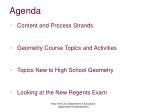Agenda
• Content and Process Strands
• Geometry Course Topics and Activities
• Topics New to High School Geometry
• Looking at the New Regents Exam

New York City Department of Education

Department of Mathematics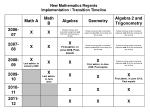New Mathematics Regents

Implementation / Transition Timeline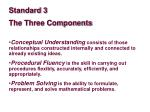Standard 3

The Three Components

• Conceptual Understanding consists of those relationships constructed internally and connected to already existing ideas.
• Procedural Fluencyis the skill in carrying out procedures flexibly, accurately, efficiently, and appropriately.
• Problem Solving is the ability to formulate, represent, and solve mathematical problems.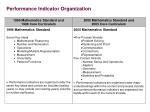Standard 3

Content and Process Strands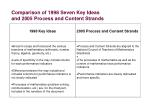Comparison of 1998 Seven Key Ideas

and 2005 Process and Content Strands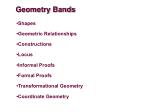Geometry Bands

•Shapes

•Geometric Relationships

•Constructions

•Locus

•Informal Proofs

•Formal Proofs

•Transformational Geometry

•Coordinate GeometryPerformanceTopicsIndicators

1 – 9 Perpendicular lines and planes

10 – 16 Properties and volumes of three- dimensional figures, including prism, regular pyramid, cylinder, right circular cone, spherePerformanceTopicsIndicators

17 – 21 Constructions: angle bisector, perpendicular bisector, parallel through a point, equilateral triangle;

22, 23 Locus: concurrence of median, altitude, angle bisector, perpendicular bisector;

compound lociPerformanceTopicsIndicators

24 – 27 Logic and proof: negation, truth value, conjunction, disjunction, conditional, biconditional, inverse, converse, contrapositive; hypothesis → conclusion

28, 29 Triangle congruence (SSS, SAS,ASA, AAS, HL) and corresponding partsPerformanceTopicsIndicators

30 – 48 Investigate, justify and apply theorems (angles and polygons):

Sum of angle measures (triangles and polygons): interior and exterior

Isosceles triangle

Geometric inequalities

Triangle inequality theorem

Largest angle, longest side

Transversals and parallel linesPerformanceTopicsIndicators

30 – 48 Investigate, justify and apply theorems (angles and polygons):

Parallelograms (including special cases), trapezoids

Line segment joining midpoints, line parallel to side (proportional)

Centroid

Similar triangles (AA, SAS, SSS)

Mean proportional

Pythagorean theorem, conversePerformanceTopicsIndicators

49 – 53 Investigate, justify and apply theorems (circles):

Chords: perpendicular bisector. relative lengths

Tangent lines

Arcs, rays (lines intersecting on, inside, outside)

Segments intersected by circle along tangents, secantsCenter of a CircleFind different ways, as many as you can, to determine the center of a circle. Imagine that you have access to tools such as compass, ruler, square corner, protractor, etc.Be able to justify that you have found the center.PerformanceTopicsIndicators

54 – 61 Transformations

Isometries (rotations, reflections, translations, glide reflections)

Use to justify geometric relationships

Similarities (dilations)

Properties that remain invariantFold and Punch

Take a square piece of paper. Fold it and make one punch so that you will have one of the following patterns when you open it.Reflective Symmetry

Translational Symmetry

Rotational Symmetry

Venn SymmetryPerformanceTopicsIndicators

62 – 68 Coordinate geometry: Distance, midpoint, slope formulas to find equations of lines perpendicular, parallel, and perpendicular bisectorPerformanceTopicsIndicators

69 Coordinate geometry: Properties of triangles and quadrilaterals

70 Coordinate geometry: Linear- quadratic systemsArea of a Triangle on a Coordinate Plane

Two vertices of a triangle are located at (0,6) and (0,12).

The area of the triangle is 12 units2.PerformanceTopicsIndicators

71 – 74 Coordinate geometry: Circles: equations, graphs (centered on and off origin)About 20% of the topics in the new Geometry course have not been addressed in previous high school courses.centroid

circumcenter

incenter of a triangle

orthocentercentroid (G)The point of concurrency of the medians of a triangle; the center of gravity in a triangle.

circumcenter (G) The center of the circle circumscribed about a polygon; the point that is equidistant from the vertices of any polygon.

incenter of a triangle (G)The center of the circle that is inscribed in a triangle; the point of concurrence of the three angle bisectors of the triangle which is equidistant from the sides of the triangle.

orthocenter (G)The point of concurrence of the three altitudes of a triangle.isometry

symmetry planeIf P′ is the image of P, and Q′ is the image of Q, then the distance from P′ to Q′ is the same as the distance from P to Q.

symmetry plane (G) A plane that intersects a three-dimensional figure such that one half is the reflected image of the other half.Geometric Relationships 1Theorems and Postulates

G.G.1 If a line is perpendicular to each of two intersecting lines at their point of intersection, then the line is perpendicular to the plane determined by them

G.G.2 Through a given point there passes one and only one plane perpendicular to a given line

G.G.3 Through a given point there passes one and only one line perpendicular to a given plane

G.G.4 Two lines perpendicular to the same plane are coplanar

G.G.5 Two planes are perpendicular to each other if and only if one plane contains a line perpendicular to the second planeG.G.1b

Study the drawing below of a pyramid whose base is quadrilateral ABCD. John claims that line segment EF is the altitude of the pyramid. Explain what John must do to prove that he is correct.G.G.3a

Examine the diagram of a right triangular prism.

Describe how a plane and the prism could intersect so that the intersection is:

a line parallel to one of the triangular bases

a line perpendicular to the triangular bases

a triangle

a rectangle

a trapezoidG.G.4b The figure below in three-dimensional space, where AB is perpendicular to BC and DC is perpendicular to BC, illustrates that two lines perpendicular to the same line are not necessarily parallel. Must two lines perpendicular to the same plane be parallel? Discuss this problem with a partner.Geometric Relationships 2More Theorems and Postulates

G.G.6 If a line is perpendicular to a plane, then any line perpendicular to the given line at its point of intersection with the given plane is in the given plane

G.G.7 If a line is perpendicular to a plane, then every plane containing the line is perpendicular to the given plane

G.G.8 If a plane intersects two parallel planes, then the intersection is two parallel lines

G.G.9 If two planes are perpendicular to the same line, they are parallelG.G.7a Examine the four figures below:

Each figure has how many symmetry planes?

Describe the location of all the symmetry planes for each figure.G.G.9a The figure below shows a right hexagonal prism.

A plane that intersects a three-dimensional figure such that one half is the reflected image of the other half is called a symmetry plane. On a copy of the figure sketch a symmetry plane. Then write a description of the symmetry plane that uses the word parallel.

On a copy of the figure sketch another symmetry plane. Then write a description that uses the word perpendicular.Geometric Relationships 3Prisms

G.G.10 The lateral edges of a prism are congruent and parallel

G.G.11 Two prisms have equal volumes if their bases have equal areas and their altitudes are equalG.G.11a

Examine the prisms below. Calculate the volume of each of the prisms. Observe your results and make a mathematical conjecture. Share your conjecture with several other students and formulate a conjecture that the entire group can agree on. Write a paragraph that proves your conjecture.Locus

G.G.21 Concurrence of medians, altitudes, angle bisectors, and perpendicular bisectors of trianglesG.G.21a

Using dynamic geometry software locate the circumcenter, incenter, orthocenter, and centroid of a given triangle. Use your sketch to answer the following questions:

Do any of the four centers always remain inside the circle?

If a center is moved outside the triangle, under what circumstances will it happen?

Are the four centers ever collinear? If so, under what circumstances?

Describe what happens to the centers if the triangle is a right triangle.Informal and Formal Proofs 1

G.G.43 Theorems about the centroid of a triangle, dividing each median into segments whose lengths are in the ratio 2:1G.G.43a

The vertices of a triangle ABC are A(4,5), B(6,1), and C(8,9). Determine the coordinates of the centroid of triangle ABC and investigate the lengths of the segments of the medians. Make a conjecture.Informal and Formal Proofs 2Similarity

G.G.46 Theorems about proportional relationships among the segments of the sides of the triangle, given one or more lines parallel to one side of a triangle and intersecting the other two sides of the triangleG.G.46a

In ΔABC , DE is drawn parallel to AC . Model this drawing using dynamic geometry software. Using the measuring tool, determine the lengths AD, DB, CE, EB, DE, and AC. Use these lengths to form ratios and to determine if there is a relationship between any of the ratios. Drag the vertices of the original triangle to see if any of the ratios remain the same. Write a proof to establish your work.Transformational Geometry

G.G.60 Similarities: observing orientation, numbers of invariant points, and/or parallelismG.G.60a

In the accompanying figure, ΔABC is an equilateral triangle. If ΔADE is similar to ΔABC, describe the isometry and the dilation whose composition is the similarity that will transform ΔABC onto ΔADE.G.G.60b

Harry claims that ΔPMN is the image of ΔNOP under a reflection over PN.. How would you convince him that he is incorrect? Under what isometry would ΔPMN be the image of ΔNOP?Calculators

Schools must make a graphing calculator available for the

exclusive use of each student while that student takes the Regents examination in Geometry.Reference Sheet

The Regents Examination in Geometry will include a reference sheet containing the formulas specified below.Core Curriculum, Sample Tasks, Glossary, Course Descriptions, Crosswalks and Other Resources:http://www.emsc.nysed.gov/3-8/guidance912.htm

New York City Department of Education

Department of Mathematics